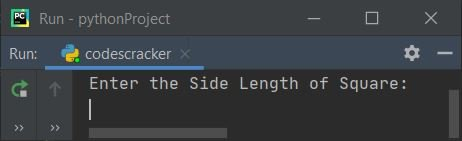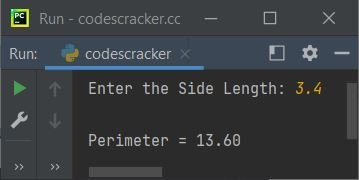# Python Program to Find Perimeter of Square

In this article, we've created some programs in Python, to find and print perimeter of a square based on the length of its side entered by user at run-time. Here are the list of programs:

• Find Perimeter of Square without Function
• Using user-defined Function
• Using class

Before starting these programs, let's first remind you about the formula used here.

### Formula to Find Perimeter of Square

To find perimeter of any square, use following formula:

`per = 4*side`

Here per indicates to perimeter, and side indicates to length of square's side.

That is, as you all knows that perimeter of a square can be calculated by adding all its four sides, or just by multiplying any side with 4.

Therefore if a square has its side length as 2 meter then its perimeter will be 2+2+2+2 or 4*2, that is equal to 16.

## Find Perimeter of Square without Function

To calculate perimeter of a square in Python, you have to ask from user to enter the side length of square, then use the above formula and calculate the perimeter as shown in the program given below:

```print("Enter the Side Length of Square: ")
s = int(input())
p = 4*s
print("\nPerimeter = ", p)```

The snapshot given below shows the initial output produced by this Python program:Now supply the input say 5 as the length of side of square and press `ENTER` key to find and print the perimeter value as shown in the snapshot given below:#### Modified Version of Previous Program

In this program, the end= is used to skip an automatic newline using print(). And {:.2f} is used with format() to print the value of p upto only two decimal places.

```print("Enter the Side Length: ", end="")
s = float(input())
p = 4*s
print("\nPerimeter = {:.2f}".format(p))```

Here is its sample run with user input 3.4 as side length of square:## Find Perimeter of Square using Function

This program does the same job as of previous, but using user-defined function named findPerSqr(). This function receives the length of any side of square as its argument, and returns the area value.

```def findPerSqr(x):
return 4*x

print("Enter the Side Length: ", end="")
s = float(input())

p = findPerSqr(s)
print("\nPerimeter = {:.2f}".format(p))```

This program produces the same output as of previous program's output.

## Find Perimeter of Square using Class

This is the last program of this article, created using class, an object-oriented feature of Python. The member function of class is accessed through its object. Therefore, we've created an object named ob and through this object, we've accessed the member function named findPerSqr() of the class CodesCracker.

```class CodesCracker:
def findPerSqr(self, x):
return 4*x

print("Enter the Side Length: ", end="")
s = float(input())

ob = CodesCracker()
p = ob.findPerSqr(s)
print("\nPerimeter = {:.2f}".format(p))```

#### Same Program in Other Languages

Python Online Test

« Previous Program Next Program »